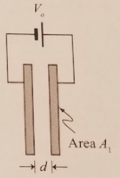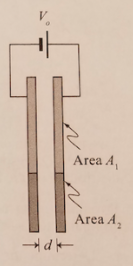# Problem: Two large flat plates are separated by a distance d. The plates are connected to a battery.a. The surface area of the face of each plate is A1. Write an expression for the capacitance in terms of A1 and d.b. A new capacitor is formed by attaching two uncharged metal plates, each with an area A2, to the capacitor as shown. The battery remains connected.i. When the new plates are attached, does the electric potential difference between the plates increase, decrease, or remain the same?ii. Write an expression for the work done by the electric field on a charge +q0, as it travels from the left plate of the capacitor to the right. Express your answer in terms of the given variables. Explain.iii. Write an expression for the magnitude and direction of the electric field between the plates. Is the magnitude of the electric field greater than, less than, or equal to the magnitude of the electric field between the plates before the new plates were attached?iv. Write an expression for the charge density on the plates of the capacitor. Is the charge density greater than, less than, or equal to the charge density on the plates before the new plates were attached? Explain

###### FREE Expert Solution

a.

The capacitance of a parallel plate capacitor:

$\overline{){\mathbf{C}}{\mathbf{=}}\frac{{\mathbf{\epsilon }}_{\mathbf{0}}\mathbf{A}}{\mathbf{d}}}$

Substituting in the equation of a parallel plate capacitor:

C = ε0A1/d

The capacitance of the capacitor is ε0A1/d

94% (192 ratings)###### Problem DetailsTwo large flat plates are separated by a distance d. The plates are connected to a battery.

a. The surface area of the face of each plate is A1. Write an expression for the capacitance in terms of A1 and d.b. A new capacitor is formed by attaching two uncharged metal plates, each with an area A2, to the capacitor as shown. The battery remains connected.

i. When the new plates are attached, does the electric potential difference between the plates increase, decrease, or remain the same?
ii. Write an expression for the work done by the electric field on a charge +q0, as it travels from the left plate of the capacitor to the right. Express your answer in terms of the given variables. Explain.
iii. Write an expression for the magnitude and direction of the electric field between the plates. Is the magnitude of the electric field greater than, less than, or equal to the magnitude of the electric field between the plates before the new plates were attached?
iv. Write an expression for the charge density on the plates of the capacitor. Is the charge density greater than, less than, or equal to the charge density on the plates before the new plates were attached? Explain